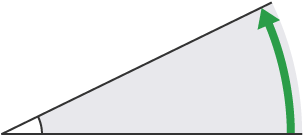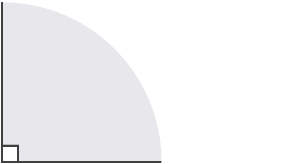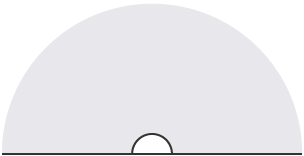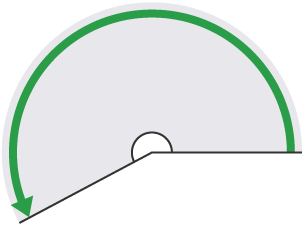Let’s do a little brain test right this moment. Without prior notice or recourse to a material on angles, can you mention the different angles there are, and what defines them?

Whether yes or no, scroll down to see how well you did or how much you need to know.

### 1. ACUTE ANGLE

This angle is less thanIn the image above, you can see that the line turns less than a right angle to get to the other line, so it must be betweenand.

### 2. RIGHT ANGLE

This angle is exactly### 3. OBTUSE ANGLE

An obtuse angle is betweenandIn this example, the angle lies somewhere betweenand, but seems slightly closer to, so you could estimate this angle to be.

### 4. ANGLE ON A STRAIGHT LINE

A straight angle or an angle on a straight line is exactly### 5. REFLEX ANGLE

This angle is betweenandIn the image above, notice how the line turns through more than two right angles, but less than three right angles, so is betweenand.

### 6. FULL TURN or COMPLETE REVOLUTION

This is exactly.